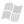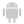WINDEVWEBDEVWINDEV MobileOthersCumulated (Property)
In french: Cumul
The Cumulated property is used to determine if the value of a column or row in Pivot Table control corresponds to a total (total at the end of a row or column). This property can only be used in the display process of a cell.
Example
// Display code of a cell in a Pivot Table control

IF COL_OrderDate_Year.Cumulated = True OR COL_Country.Cumulated = True THEN
fntFont is Font
// We are positioned on a total row/column
fntFont.Name = "Trebuchet MS"
fntFont.Size = 8
fntFont.Color = Black
fntFont.Bold = True
fntFont.Italic = True

// Changes the background color of totals
VAL_TO.BackgroundColor = YellowToolhelp
VAL_Qty.BackgroundColor = YellowToolhelp

// Changes the font
VAL_TO.Font = fntFont
VAL_Qty.Font = fntFont
ELSE
IF VAL_TO <= 500 _AND_ VAL_TO > 0 THEN
VAL_TO.BackgroundColor = PastelRed
END
IF VAL_TO >= 30000  THEN
VAL_TO.BackgroundColor = PastelGreen
END
END
Syntax
<Result> = <Row or column header>.Cumulated
<Result>: Boolean
• True if the row or column corresponds to a total,
• False otherwise.
<Row or column header>: Control name
Remarks
You can also find out whether the value of a row or column found in a Pivot Table control corresponds to a total by checking the value of the row or column.
For a total:
• the value of the row or column corresponds to "*".
• the Cumulated property is set to True.
// Display code of a cell in a Pivot Table control

IF COL_OrderDate_Year.Cumulated = "*" OR COL_Country.Cumulated = "*" THEN
fntFont is Font
// We are positioned on a total row/column
fntFont.Name = "Trebuchet MS"
fntFont.Size = 8
fntFont.Color = Black
fntFont.Bold = True
fntFont.Italic = True

// Changes the background color of totals
VAL_TO.BackgroundColor = YellowToolhelp
VAL_Qty.BackgroundColor = YellowToolhelp

// Changes the font
VAL_TO.Font = fntFont
VAL_Qty.Font = fntFont
ELSE
IF VAL_TO <= 500 _AND_ VAL_TO > 0 THEN
VAL_TO.BackgroundColor = PastelRed
END
IF VAL_TO >= 30000  THEN
VAL_TO.BackgroundColor = PastelGreen
END
END
Related Examples:Training (WINDEV): WD PivotTable [ + ] This example presents the use of the Pivot Table control.This Pivot Table control is used to display dynamic statistics while grouping the data.These statistics are calculated form the database.
Minimum version required
• Version 18